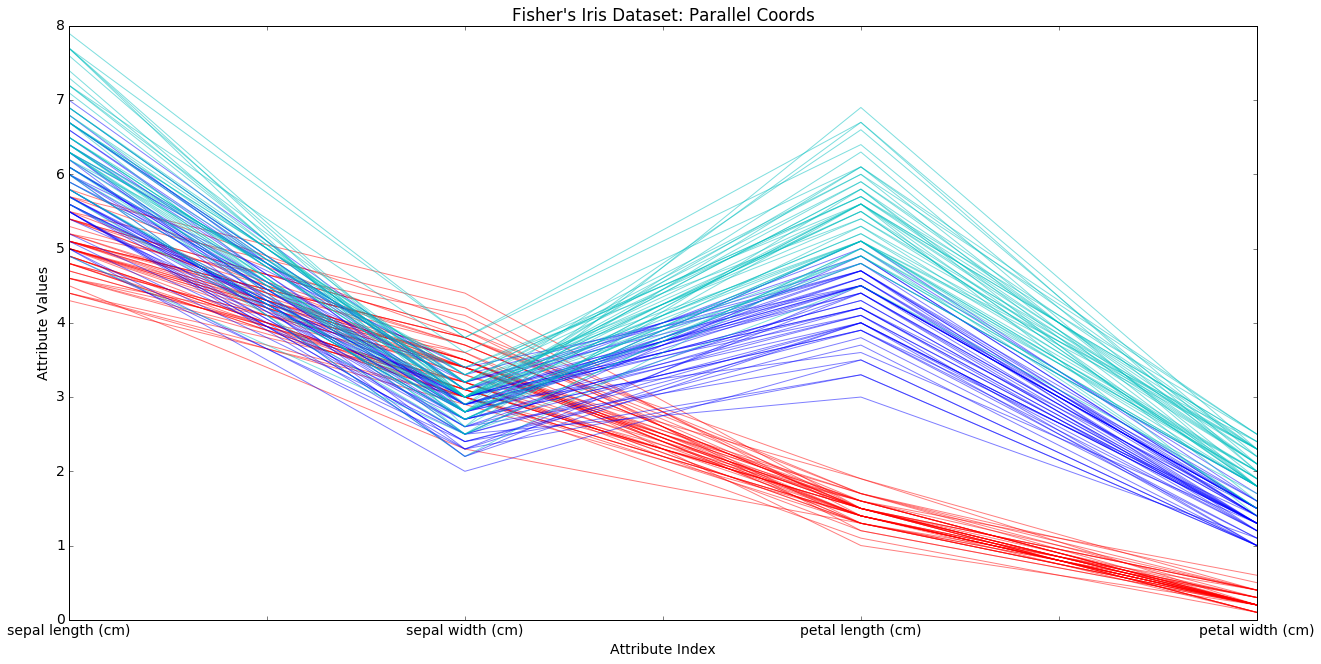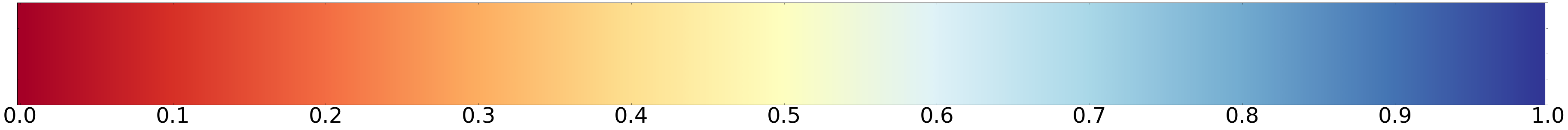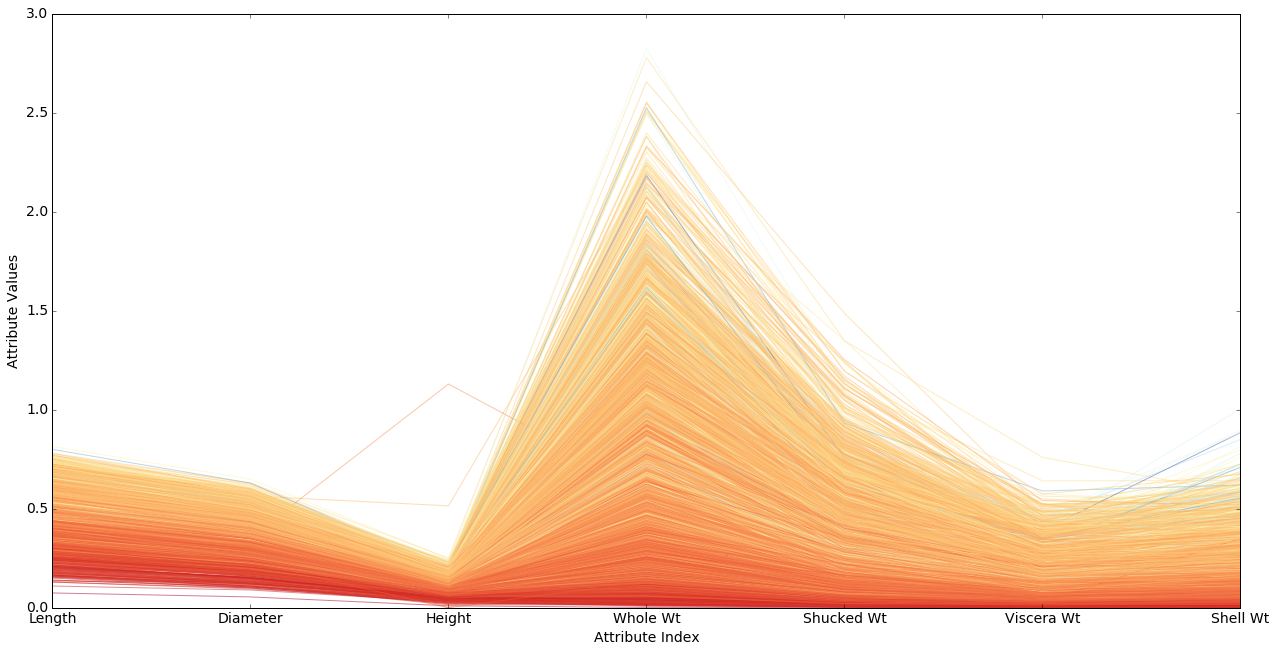# 9.3. Color¶

## 9.3.1. Using Colors and Color Maps I¶

### 9.3.1.1. 1. Parallel plots: Fisher’s irises¶

```%matplotlib inline

import pandas as pd
from pandas import DataFrame
import matplotlib.pyplot as plt
from sklearn import datasets
import matplotlib as mpl
import numpy as np

X = iris.data[:,:4]
col_labels = iris.feature_names
df = pd.DataFrame(X, columns=col_labels)
```

Here are the attributes the `sklearn` data object has. The attributes are common to many of the `sklearn` data sets.

```iris.viewkeys()
```

Fisher’s data sets contains data on 3 species of iris.

```iris.target_names
```
```iris.target
```

`iris.target` is a 1D numpy array with the 3 targets assigned as ints (0,1,2) and iris.data is a numpy array with the 4 attributes.

```iris.feature_names
```

The data contains measurements for 4 morphological (shape-related) attributes of irises.

```print iris.DESCR
```

Next we draw what is known as a parallel coordinates plot of the data. See the reference section for more on such plots.

The x-axis is for the 4 morphological attributes, the y-axis for their values. We draw lines containing the values of the attributes for all data points, choosing the line color according to class. We first draw the line for one particular data point, iris 0, the first iris among our 150 exexamplar. By itself it tells us little.

```plt.figure(figsize=(20,10))
mpl.rcParams['xtick.labelsize'] = 14
mpl.rcParams['font.size'] = 14

#Choose a color
if iris.target == 0:
pcolor = "r"
elif iris.target == 1:
pcolor = "b"
elif iris.target == 2:
pcolor = "c"
# identify the row to be plotted.
dataRow = df.iloc[0,:]
# `pandas` plot method uses matplotlib under the hood.
dataRow.plot(color=pcolor)
plt.title("One example iris")
plt.xlabel("Attribute Index")
plt.ylabel(("Attribute Values"))
## Draw
#plt.show()
```
```<matplotlib.text.Text at 0x118828f10>
```In the next cell we draw all 150 data lines. What we are doing is visualizing points in a 4-dimensional space.

We are looking for regularities within the classes, and there are some. We see condirable separation of the red class on the petal attributes, and a trend toward separation of the blue and green class on sepal attributes. But note there is considerable overlap as well. This is part of why those classes are harder to separate. Note also the the blue and greem classes move in parallel; they rise and fall together. This, together with the overlap, is why they are harder to separate.

Nevertheless, what we are seeing is consierable structure in the way the attributes are distributed. Compare the next example.

```plt.figure(figsize=(20,10))
mpl.rcParams['xtick.labelsize'] = 14
mpl.rcParams['font.size'] = 14

for i in range(len(X)):
#assign color based on Fisher's classes 0, 1 or 2
if iris.target[i] == 0:
pcolor = "r"
elif iris.target[i] == 1:
pcolor = "b"
elif iris.target[i] == 2:
pcolor = "c"

#plot rows of data as if they were series data
dataRow = df.iloc[i,:]
dataRow.plot(color=pcolor, alpha=0.5)

### Add some essentials to the plot before drawing
plt.title("Fisher's Iris Dataset: Parallel Coords")
plt.xlabel("Attribute Index")
plt.ylabel(("Attribute Values"))
## Draw
#plot.show()
```
```<matplotlib.text.Text at 0x11890f550>
```### 9.3.1.2. 2. Abalone parallel plots: Regression example (age prediction)¶

Adapted from code by Mike Bowles.

Fist load the abalone data. This is a regression problem, predicting a continuous value (age) from continuous independent variables.

```import pandas as pd
import numpy as np
from pandas import DataFrame
import matplotlib as mpl
import matplotlib.pyplot as plot
from math import exp
target_url = ("http://archive.ics.uci.edu/ml/machine-"
"learning-databases/abalone/abalone.data")
abalone.columns = ['Sex', 'Length', 'Diameter', 'Height',
'Whole Wt', 'Shucked Wt',
'Viscera Wt', 'Shell Wt', 'Rings']
#get summary to use for scaling
summary = abalone.describe()
# We are going to use the max/min value for 'age' (number of rings
# to normalize ages)
minRings = summary.iloc[3,7]
maxRings = summary.iloc[7,7]
meanRings = summary.iloc[1,7]
sdRings = summary.iloc[2,7]

plt.clf()
```
```<matplotlib.figure.Figure at 0x1188f6210>
```

The issue here is that since we are trying to predict age, so we don’t have discrete classes to color. We’re using various weight and length measurements to predict the age of the abalone, and using the number of rings (stored in the `target` array) as our representation of age.

To color this data, then, we want a color map; a color map chooses from a range of colors based on a number it is given.

We’ll use a particular `matplotlib` color map called `RdYlBu`. Here’s how it looks.

```def draw_colormap (cmap_name,width=60,height=4,fontsize=50):
"""
cmap_name is a string.
"""
fig = plt.figure(figsize=(width,height))
ax = plt.gca()
mpl.rcParams['xtick.labelsize'] = fontsize
mpl.rcParams['font.size'] = fontsize
# Divide the interval from 0 to 1 into 256 parts.
# The imshow function actually displays images,
# but you can pass it an array.
# In either case, the function wants 2D info.
# We give it an array with 2 rows,
# make the top half and bottom half  of the image the same.
# We'll vertically stretch this very skinny image with aspect 'auto'.
# And the value of gradient will change the color value from left to right.
# Show the number values associated with color on x-axis.
# The xvalues that imshow uses are pixel numbers, integers from 0 to 256.
ax.set_xticks(np.linspace(0,256,11))
# We'll label those with the inputs to the color map, numbers from 0 to 1
ax.set_xticklabels(np.linspace(0,1,11))
ax.set_yticklabels([])

# Put in an color map name you like here.
cmap_name = 'RdYlBu'
draw_colormap(cmap_name)
```The `RdYlBu` color map wants numbers between 0 and 1 and will make lower numbers in that range reddish, mid range number yellowish, and higher numbers bluish, basically the range from hot to cool colors.

The cell below will draw a parallel plot for the abalone data using these color values. Patience. This may take a while.

1. The variable `labelColor` in the code below is the normalized age of the abalone exemplar we’re drawing the line for. We pass this normalized age to our color map, which converts the age into a color. The yoiungest abalone should be red, the oldest blue, allthe others some color in between, where “in between” means according to the color map shown above. We see that the young (reddest) abalone are concentrated in the lower part of the plot, indicating, not surprisingly, that they have lower mesasurements on all the attributes.

2. There are many different colormaps (see matplotlib colormaps demo). The `RdYlBu` map is what’s called a divergent color map in the `mpl` docs:

```These colormaps have a median value (usually light in color) and vary
smoothly to two different color tones at high and low values. Diverging
colormaps are ideal when your data has a median value that is significant
(e.g.  0, such that positive and negative values are represented by
different colors of the colormap).
```

Before mapping an age to a color the code below first normalizes the age. We map the age to a number between 0 and 1 which represents what proportion of the way this age is from the minimum age to the maximum age. 3. We leave out non-numerical attributes. In this case, there’s only 1, the `Sex` column.

```plt.figure(figsize=(20,10))
mpl.rcParams['xtick.labelsize'] = 14
mpl.rcParams['font.size'] = 14
for i in range(nrows):
dataRow = abalone.iloc[i,1:8]
# Getting the age info and "normalizing" it.
labelColor = (abalone.iloc[i,8] - minRings) / (maxRings - minRings)
# You can try this instead.  It doesnt help much.
#labelColor = centered_age(abalone.iloc[i,8])
#Choose color for this line based on how old the exemplar is
dataRow.plot(color=plot.cm.RdYlBu(labelColor), alpha=0.5)
#Use the dsame color for every exemplar. Dull.
#dataRow.plot(color=plot.cm.RdYlBu(.1), alpha=0.5)

plot.xlabel("Attribute Index")
plot.ylabel(("Attribute Values"))
plot.show()

#renormalize using mean and standard variation, then compress
# with logit function
```Note that almost all the colors fall in the red-yellow range. That means the right hand half of the color map shown in the cell above is almost entirely missing.

The problem is that our normalized data numbers don’t make very good use of the color map. The normalized age values go from about 0 to 1 as they should, but there are lots of abalone crowded into the reddish range and very few getting bluish colors. That means we’re wasting most of our color bandwidth.

What we need to do is recenter our data so that the most heavily populated part of the age range gets mapped to .5.

### 9.3.1.3. 3. Abalone data using inverse logit transform¶

So we do a very common data transformation. We do the logistic function, or `inverse-logit` of the normalized ages:

\$ x ({1 + e{-x}}){-1} \$

This maps every centered age to a number between 0 and 1.

Note that we’re not going to change the values of the attributes in any way, just the way the plot lines are interpreted as colors. We’ll get the same overall silhouette for the plot.

```def norm_age (x):
"""
This is what we were doing in the first plot.
"""
return (x - minRings) / (maxRings - minRings)

def centered_age (x):
"""
Here's the first step in the new mapping. Center the data.

Distance from mean divided by STD => distance above/below mean in
STD units.
"""
return (x - meanRings)/sdRings

def inverse_logit (x):
return 1./(1 + np.exp(-x))
```

Here are some numbers to give you an idea of what `logit` does to the ages. The firat number is the age and the last number is the count of the number of abalone exemplars of that age. In between are the normalized ages and the inverse logit of the ages. The most heavily populated part of the distribution is age 9 with a normalized value of .29 and a logit value of .43, so that very popular age has been moved to a value close to .5. So we get yellow for that age, and evenly balanced blues and reds for the ages on either side.

```from collections import Counter
# get the 9th column of the data, with the age info
ages = abalone.iloc[:,8]
(amax,amin) = ages.max(),ages.min()
# Keep track of how many exemplars there are of each age.
ctr = Counter(ages)
for i in range(amin,amax):
print '{0:>2}  {1: .4f}   =>   {2:.4f}   {3:>3d}'.format(i, norm_age(i),inverse_logit(centered_age(i)),ctr[i])
```
``` 1   0.0000   =>   0.0589     1
2   0.0357   =>   0.0787     1
3   0.0714   =>   0.1043    15
4   0.1071   =>   0.1370    57
5   0.1429   =>   0.1780   115
6   0.1786   =>   0.2279   259
7   0.2143   =>   0.2870   391
8   0.2500   =>   0.3544   568
9   0.2857   =>   0.4281   689
10   0.3214   =>   0.5051   634
11   0.3571   =>   0.5819   487
12   0.3929   =>   0.6550   267
13   0.4286   =>   0.7213   203
14   0.4643   =>   0.7792   126
15   0.5000   =>   0.8280   103
16   0.5357   =>   0.8678    67
17   0.5714   =>   0.8995    58
18   0.6071   =>   0.9243    42
19   0.6429   =>   0.9433    32
20   0.6786   =>   0.9578    26
21   0.7143   =>   0.9687    14
22   0.7500   =>   0.9769     6
23   0.7857   =>   0.9829     9
24   0.8214   =>   0.9874     2
25   0.8571   =>   0.9907     1
26   0.8929   =>   0.9932     1
27   0.9286   =>   0.9950     2
28   0.9643   =>   0.9963     0
```
```plt.figure(figsize=(20,10))
mpl.rcParams['xtick.labelsize'] = 14
mpl.rcParams['font.size'] = 14

# When experimenting with drawing params, draw only a subset of
# the rows, to reduce waittime
# nrows = 100
# Or set it back to what it should be
nrows = len(abalone.index)

for i in range(nrows):
dataRow = abalone.iloc[i,1:8]
# Center the data point
normTarget = (abalone.iloc[i,8] - meanRings)/sdRings
# Do inverse logit
labelColor = 1.0/(1.0 + exp(-normTarget))
dataRow.plot(color=plot.cm.RdYlBu(labelColor), alpha=0.5)

plot.xlabel("Attribute Index",fontsize=16)
plot.ylabel("Attribute Values",fontsize=16)
#plot.show()
```
```<matplotlib.text.Text at 0x119c37a10>
```Now we can see the picture is considerable richer than it looked before. There are a large number of cases of older abalone with smaller than expected measurements (the darker stripes in various strata), and it looks as if those exemplars are consistently displaced. That is, if you have a smaller than average whole weight for your age, then you are likely to have a smaller than average height. If that’s true of all the attributes, the age of those exemplars is going to be hard to predict.

### 9.3.1.4. When it doesn’t work¶

Back to classification instead of regression.

Rock v. Mines data. 2 classes, two colors. Showing only 8 of 60 attributes below.

What goes wrong?

```__author__ = 'mike_bowles'
import pandas as pd
from pandas import DataFrame
import matplotlib.pyplot as plot
target_url = ("https://archive.ics.uci.edu/ml/machine-learning-"
"databases/undocumented/connectionist-bench/sonar/sonar.all-data")
plt.figure(figsize=(20,10))
mpl.rcParams['xtick.labelsize'] = 14
mpl.rcParams['font.size'] = 14

#read rocks versus mines data into pandas data frame
```
```<matplotlib.figure.Figure at 0x118849450>
```
```print len(rocksVMines)
print rocksVMines.columns
# V60, the last column, is non-numerical; it's the one we're predicting
print set(rocksVMines.iloc[:,60].values)
```
```208
Index([u'V0', u'V1', u'V2', u'V3', u'V4', u'V5', u'V6', u'V7', u'V8', u'V9',
u'V10', u'V11', u'V12', u'V13', u'V14', u'V15', u'V16', u'V17', u'V18',
u'V19', u'V20', u'V21', u'V22', u'V23', u'V24', u'V25', u'V26', u'V27',
u'V28', u'V29', u'V30', u'V31', u'V32', u'V33', u'V34', u'V35', u'V36',
u'V37', u'V38', u'V39', u'V40', u'V41', u'V42', u'V43', u'V44', u'V45',
u'V46', u'V47', u'V48', u'V49', u'V50', u'V51', u'V52', u'V53', u'V54',
u'V55', u'V56', u'V57', u'V58', u'V59', u'V60'],
dtype='object')
set(['R', 'M'])
```

Making use of the very useful `pandas` `describe` method on dataframes, which prints out a number of statistics about the data.

```summary = rocksVMines.describe()
rocksVMines.describe()
```
V0 V1 V2 V3 V4 V5 V6 V7 V8 V9 ... V50 V51 V52 V53 V54 V55 V56 V57 V58 V59
count 208.000000 208.000000 208.000000 208.000000 208.000000 208.000000 208.000000 208.000000 208.000000 208.000000 ... 208.000000 208.000000 208.000000 208.000000 208.000000 208.000000 208.000000 208.000000 208.000000 208.000000
mean 0.029164 0.038437 0.043832 0.053892 0.075202 0.104570 0.121747 0.134799 0.178003 0.208259 ... 0.016069 0.013420 0.010709 0.010941 0.009290 0.008222 0.007820 0.007949 0.007941 0.006507
std 0.022991 0.032960 0.038428 0.046528 0.055552 0.059105 0.061788 0.085152 0.118387 0.134416 ... 0.012008 0.009634 0.007060 0.007301 0.007088 0.005736 0.005785 0.006470 0.006181 0.005031
min 0.001500 0.000600 0.001500 0.005800 0.006700 0.010200 0.003300 0.005500 0.007500 0.011300 ... 0.000000 0.000800 0.000500 0.001000 0.000600 0.000400 0.000300 0.000300 0.000100 0.000600
25% 0.013350 0.016450 0.018950 0.024375 0.038050 0.067025 0.080900 0.080425 0.097025 0.111275 ... 0.008425 0.007275 0.005075 0.005375 0.004150 0.004400 0.003700 0.003600 0.003675 0.003100
50% 0.022800 0.030800 0.034300 0.044050 0.062500 0.092150 0.106950 0.112100 0.152250 0.182400 ... 0.013900 0.011400 0.009550 0.009300 0.007500 0.006850 0.005950 0.005800 0.006400 0.005300
75% 0.035550 0.047950 0.057950 0.064500 0.100275 0.134125 0.154000 0.169600 0.233425 0.268700 ... 0.020825 0.016725 0.014900 0.014500 0.012100 0.010575 0.010425 0.010350 0.010325 0.008525
max 0.137100 0.233900 0.305900 0.426400 0.401000 0.382300 0.372900 0.459000 0.682800 0.710600 ... 0.100400 0.070900 0.039000 0.035200 0.044700 0.039400 0.035500 0.044000 0.036400 0.043900

8 rows × 60 columns

Note that the summary object returned by `rocksVMines.describe()` is itself a data frame, and can be used to find out a statistic about an attribute. For example, to find out the mean of `V3`, do

```summary.iloc[1,3]
```
```0.053892307692307684
```

Note the maxes, means, stds, and medians rise steadily as we move rightwards, but by the time we get to `V50` they’re down again. Hmm.

It turns out this is sonar Time Series data, meaning we’re looking at data points taken from successive moments in time. The data is also cyclic, and it rises and falls, wavelike.

```plt.figure(figsize=(20,10))
mpl.rcParams['xtick.labelsize'] = 14
mpl.rcParams['font.size'] = 14
for i in range(208):
#assign color based on color based on "M" or "R" labels
if rocksVMines.iat[i,60] == "M":
pcolor = "red"
else:
pcolor = "blue"

#plot just 20 rows of data as if they were series data
dataRow = rocksVMines.iloc[i,0:8]
dataRow.plot(color=pcolor, alpha=0.5)

plot.xlabel("Attribute Index")
plot.ylabel(("Attribute Values"))
#plot.show()
```
```<matplotlib.text.Text at 0x12affd6d0>
```It looks as if there may be a tendency for very low values of most of the attributes to be rocks (blue), but there’s considerable mixing of the classes for many values and many attributes. In other words, it’s a mess.

### 9.3.1.5. Homework¶

Here is some wine data. The last column is what’s to be predicted, the quality. For some reason, the quality of the wine is represented as an integer from 3 to 8, with an average value of 5.6. So bad wine gets a score of 3, and the best wine a score of 8.

```target_url = ("http://archive.ics.uci.edu/ml/machine-"
"learning-databases/wine-quality/winequality-red.csv")

#print column names in order to have the full versions
print(wine.columns)

#change column names to shorter ones to fit graph
wine.columns = ['fixAcid', 'volAcid', 'citAcid',
'resSugr', 'chlor', 'frSO2', 'totSO2',
'dens', 'pH', 'sulpha', 'alcohol', 'quality']

#generate statistical summaries
summary = wine.describe()
nrows = len(wine.index)
tasteCol = len(summary.columns)
meanTaste = summary.iloc[1,tasteCol - 1]
sdTaste = summary.iloc[2,tasteCol - 1]
nDataCol = len(wine.columns) -1
```
```Index([u'fixed acidity', u'volatile acidity', u'citric acid',
u'residual sugar', u'chlorides', u'free sulfur dioxide',
u'total sulfur dioxide', u'density', u'pH', u'sulphates', u'alcohol',
u'quality'],
dtype='object')
```
```wine.describe()
```
fixAcid volAcid citAcid resSugr chlor frSO2 totSO2 dens pH sulpha alcohol quality
count 1599.000000 1599.000000 1599.000000 1599.000000 1599.000000 1599.000000 1599.000000 1599.000000 1599.000000 1599.000000 1599.000000 1599.000000
mean 8.319637 0.527821 0.270976 2.538806 0.087467 15.874922 46.467792 0.996747 3.311113 0.658149 10.422983 5.636023
std 1.741096 0.179060 0.194801 1.409928 0.047065 10.460157 32.895324 0.001887 0.154386 0.169507 1.065668 0.807569
min 4.600000 0.120000 0.000000 0.900000 0.012000 1.000000 6.000000 0.990070 2.740000 0.330000 8.400000 3.000000
25% 7.100000 0.390000 0.090000 1.900000 0.070000 7.000000 22.000000 0.995600 3.210000 0.550000 9.500000 5.000000
50% 7.900000 0.520000 0.260000 2.200000 0.079000 14.000000 38.000000 0.996750 3.310000 0.620000 10.200000 6.000000
75% 9.200000 0.640000 0.420000 2.600000 0.090000 21.000000 62.000000 0.997835 3.400000 0.730000 11.100000 6.000000
max 15.900000 1.580000 1.000000 15.500000 0.611000 72.000000 289.000000 1.003690 4.010000 2.000000 14.900000 8.000000

As you can see the various columns have huge variety in the means, so it is helpful to center them all and put them in STD units. This means the maximums in all the columns will all be of comparable size. The next cell defines a copy of the wine data frame called `wineNormalized` in which the values of all the attributes have all been centered.

```wineNormalized = wine
ncols = len(wineNormalized.columns)

for i in range(ncols):
mean = summary.iloc[1, i]
sd = summary.iloc[2, i]
wineNormalized.iloc[:,i:(i + 1)] = \
(wineNormalized.iloc[:,i:(i + 1)] - mean) / sd
```

Your job is to do a parallel plot of the wine data. Use `logit` as in the second abalone plot. You can try both `wine` and `wineNormalized`, but do at least the latter.